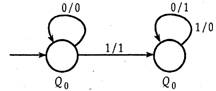Related Articles
GATE | GATE-CS-2005 | Question 63
• Last Updated : 13 Sep, 2014

The following diagram represents a finite state machine which takes as input a binary number from the least significant bit.Which one of the following is TRUE?
(A) It computes 1’s complement of the input number
(B) It computes 2’s complement of the input number
(C) It increments the input number
(D) It decrements the input number

Answer: (B)

Explanation: The given finite state machine takes a binary number from LSB as input.

The given FSM remains unchanged till first ‘1’ . After that it takes 1’s complement of rest of the input string.

We assume the input string to be ‘110010’ .
Thus, according to the FSM, output is ‘001110’ .

2’s complement of ‘110010’ = 1’s complement of ‘110010’ + 1 = 001101 + 1 = 001110
Thus, the FSM computes 2’s complement of the input string.

Hence, option (B) is correct.

Please comment below if you find anything wrong in the above post.

Quiz of this QuestionMy Personal Notes arrow_drop_up